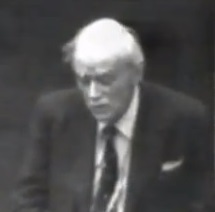Animated Physics - Paul Dirac Live

Spinning Bloch Spheres
Larmor Frequency
Rabi Oscillations
Qantum Spin States

 Paul Dirac Live!Dirac himself discussing the history of quantum mechanics and source of his discovery of the "Dirac Equation". He shows how the Poisson Bracket matches his commutators and expands on the Klien-Gordon equation for spin.

Dirac Lecture 1 (of 4) - Quantum Mechanics

 00:00 Success of Rutherford and Bohr orbit theory 00:38 Rutherford model, positive nucleus with electrons around it 00:50 Bohr found laws regarding motion of electrons - normal Newtonian classical laws but ignore radiation emission 01:50 Resolves why do electrons not fall into nucleus and emit radiation 02:20 Bohr added quantum conditions related to Planck constant 02:30 Electron jumps from orbit to orbit and emits quantum of radiation 03:05 Frequency related to energy through Planck constant 03:30 Successful in describing single electron systems, hydrogen and alkali elements 04:15 Bohr orbit theory revelation and acceptance 04:50 Sommerfeld added Hamiltonian variables of coordinates and momentum 05:33 Lagrange formulation of any function of position and velocities 05:50 Hamilton, 100 yrs earlier, replacing coordinates with momentum led to symmetry 07:00 Studied Hamiltonians by reading Whitaker and invariance of transformations 07:47 Difficulties occur on interaction of orbits 08:00 Helium spectrum appears as 2 different spectra with rare interaction 08:30 Two kinds of helium - para and ortho 09:00 Heisenberg in 1925 introduces matrix mechanics 11:00 Understands importance of Heisenberg method 11:30 Bohr orbits not physical, cannot observer electrons, observations always involve 2 states 12:20 Concentrate on observations and represent particles as matrix arrays 13:30 Heisenberg handles matrices mathematically - multiplication does not compute, ie. A*B <> B*A 15:20 Dirac concentrates on non-commutation and adds to Newtonian mechanics 16:50 Describes discovery that non-commutation and Poisson bracket are same thing in 1925 20:20 Definition of Poisson bracket - p's and q's are Hamiltonian coordinates and momentum 22:20 Equation shows direct relationship and describes path from any classical system to new mechanics 23:08 Heisenberg (with Born) showed same thing through degrees of freedom 24:20 Equations of motion - hamiltonian q/p variables represent total energy 25:40 Schrodinger's quantum mechanics - equivalent to Heisenberg theory, only needed to add wave function 27:50 Matrices associated with 2 atomic states, wave function represents an atomic state 28:08 Wave funtion Psi = function of particle coordinates x1, x2, x3 and time 28:30 Subject to wave equation where some operators produce zero 29:00 de Broglie's free particle wave equation shows momentum and energy equations are relativistic 31:00 Schrodinger applied de Broglie's free particle in an electric field 31:32 Hydrogen energy level calculation is wrong due to lack of term for spin component 32:47 Non-relativistic approximation gave correct Hydrogen energy levels 34:25 Wave equation is the Klein-Gordon equation 35:32 Schrodinger adds to Heisenberg theory to give single state 35:48 Heisenberg matrices correspond to linear operators applied to wave functions 36:05 Commutation relation between momentum and coordinate variables are same in 2 theories 37:50 Quantum mechanics more general then classical mechanics 38:35 Quantum mechanics can use any functions to give equations of motion for any hamiltonian variables 39:30 Ie., 3 componenets of spin s1, s2, s3 that satisfy same conditions as orbital angular momentum 41:06 Spin variables not expressable as q's and p's at all times 42:10 Use groups, SU2 or SU3 to describe new particles being discovered 43:22 Used to study system with many particles - ie. lots of electrons 44:00 Symmetrical vs anti-symmetrical wave function permutation operators expands quantum mechanics 45:18 Explained the spectrum of ortho and para helium 45:47 Operators can be used for absorption and emission of particles, number of particles is not conserved 46:20 Led to Fields in Quantum Mechanics, allows for transformation of general dynamical variable 46:54 Average value of dynamic variable and their powers allows you to calculate probability of value 48:10 Formula for probability of particular value is square of modulus of wave function 49:00 P's and Q's do not commute so one cannot be calculated from the other 50:15 Probability is best we can do with existing quantum mechanic formulas - does god play dice? 51:05 Bohr correct on existing quantum mechanics, but fundamental difficulties exist 52:00 People forget problem of interacting Bohr orbitals, but are too complacent in accepting current QM 52:35 Final answer will involve large basic change in ways of thinking 52:50 Who knows what will happen to determinism, we cannot go back to classical physics

Learn more...

 AnimatedPhysics.com © 2013 Contact Us